﻿ An Absorbing Markov Chain Model for Problem-Solving

### An Absorbing Markov Chain Model for Problem-Solving

Michael Gr. Voskoglou

American Journal of Applied Mathematics and Statistics

## An Absorbing Markov Chain Model for Problem-Solving

Michael Gr. VoskoglouDepartment of Mathematical Sciences, School of Technological Applications, Graduate Technological Educational Institute (T. E. I.) of Western Greece, Patras, Greece

### Abstract

In the present paper an absorbing Markov Chain model is developed for the description of the problem-solving process and through it a measure is obtained for problem-solving skills. Examples are also presented illustrating the model’s applicability in practice.

• Michael Gr. Voskoglou. An Absorbing Markov Chain Model for Problem-Solving. American Journal of Applied Mathematics and Statistics. Vol. 4, No. 6, 2016, pp 173-177. http://pubs.sciepub.com/ajams/4/6/2
• Voskoglou, Michael Gr.. "An Absorbing Markov Chain Model for Problem-Solving." American Journal of Applied Mathematics and Statistics 4.6 (2016): 173-177.
• Voskoglou, M. G. (2016). An Absorbing Markov Chain Model for Problem-Solving. American Journal of Applied Mathematics and Statistics, 4(6), 173-177.
• Voskoglou, Michael Gr.. "An Absorbing Markov Chain Model for Problem-Solving." American Journal of Applied Mathematics and Statistics 4, no. 6 (2016): 173-177.

 Import into BibTeX Import into EndNote Import into RefMan Import into RefWorks

1
Prev Next

### 1. Introduction

As the human society moved from an industrial to a knowledge society , it can be argued that the nature of many problems has been changed and new problems have arisen which may require a different approach to overcome them. Educational institutions and governments have recognized long ago the importance of Problem–Solving (PS) and volumes of research have been written about it. Universities and other higher learning institutions are entrusted with the task of producing graduates that have higher order PS skills among other skills.

Mathematics by its nature is a subject whereby PS forms its essence. In Voskoglou  we have examined the role of the problem in learning mathematics and we have attempted a review of the evolution of research on PS in mathematics education from the time that Polya  presented his first ideas on the subject until today.

In contrast to the earlier work on PS, which was focused mainly on analyzing the PS process and on describing the proper heuristic strategies to be used in each of its steps, the research has turned today mainly on solvers’ behavior and required attributes during the PS process; e. g. Multidimensional PS Framework (MPSF) of Carlson and Bloom  presented in 2005, Schoenfeld’s theory of Goal-Directed Behavior  in 2010, etc.

Note that the main steps of the MPSF are actually the same to the steps of Schoenfeld’s Expert Performance Model (EPM) for PS  presented in 1980; only their names are stated differently. However, there exists a basic difference between these two models. In fact, while in the MPSF the emphasis is turned to the solver’s behavior and required attributes, the EPM is oriented towards the PS process by describing the proper heuristic strategies that may be used at each step of the process.

In an earlier paper  Voskoglou and Perdikaris introduced a Markov Chain (MC) model for PS based on EPM. In the present paper a similar model is introduced on the main steps of the MPSF and through this a measure is obtained for PS skills.

The rest of the paper is formulated as follows: In Section 2 a brief account of the MPSF is presented followed by a flow-diagram of the PS process. In Section 3 basic conclusions from the theory of the finite MCs are stated, which are necessary for the construction of the MC model for PS. The model is developed in Section 4, while in Section 5 examples are presented illustrating its use in practice. Finally, Section 6 is devoted to the final conclusions and some hints for future research.

### 2. The MPSF of Carlson and Bloom

Carlson and Bloom  drawing from the large amount of literature related to PS developed a broad taxonomy to characterize major PS attributes that have been identified as relevant to PS success. This taxonomy gave genesis to their MPSF model, which includes the following steps: Orientation, Planning, Executing and Checking.

It has been observed that once the solvers oriented themselves to the problem space, the plan-execute-check cycle was usually repeated through out the remainder of the solution’s process; only in a few cases a solver obtained the solution of a problem by making this cycle only once. Thus embedded in the framework are two cycles, one cycling forward and one cycling back, each of which includes the three out of the four steps, i.e. planning, executing and checking (, Figure 1).

It has been also observed that, when contemplating various solution approaches during the planning step of the PS process, the solvers were at times engaged in a Conjecture – Imagine - Evaluate (accept/reject) sub-cycle. Therefore, apart of the two main cycles embedded in the MPSF, it is also the above sub-cycle, which is connected to the step of planning (, Figure 1).

Let us denote by Si, i = 1, 2, 3, 4, 5, the steps of orientation, planning, conjecture – imagine – evaluate, executing and checking respectively. Then, the flow-diagram of the PS process is shown in Figure 1.Download asVeiw figureFigures index
Figure 1. The flow-diagram of the PS process

In fact, a solver, who faces difficulties at step S2, proceeds to S3. From there, if the difficulties are surpassed, he/she returns to S2 to continue the process. Otherwise he/she returns to the staring step S1 searching for additional information from problem’s data that possibly has been elapsed at first glance. The same circle may be repeated several times.

### 3. Finite Markov Chains

The theory of MCs offers ideal conditions for the study and mathematical modelling of a certain kind of situations depending on random variables. Roughly speaking, a MC is a stochastic process that moves in a sequence of steps (phases) through a set of states and has a “one-step memory”, i.e. the probability of entering a certain state in a certain step, although in practice may not be completely independent of previous steps, depends at most on the state occupied in the previous step. This is known as the Markov property.

The basic concepts of MC were introduced by A. Markov in 1907 on coding literary texts. Since then the MC theory was developed by a number of leading mathematicians, such as A. Kolmogorov, W. Feller etc. However, only from the 1960’s the importance of this theory to the Natural, Social and most of the other Applied Sciences has been recognized [1, 3, 9, 13], etc.

When the set of its states is a finite set, then we speak about a finite MC. For general facts on finite MC we refer to the classical on the subject book of Kemeny & Snell . Let us consider a finite MC with n states, say s1, s2,…, sn, where n is a non negative integer, n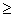2. Denote by pij the transition probability from state si to state sj , i, j = 1, 2,…, n ; then the matrix A= [pij] is called the transition matrix of the MC. Since the transition from a state to some other state (including itself) is the certain event, we have that

pi1 + pi2 +….. + pin = 1, for i=1, 2, …, n.

The row-matrix Pk = [p1(k) p2(k)… pn(k)], known as the probability vector of the MC, gives the probabilities pi(k) for the MC to be in state i at step k , for i = 1, 2,…., n and k = 0, 1, 2,…. We have again that p1(k) + p2(k) + …. + pn(k) =1.

It is well known that Pk+1= Pk A, for all non negative integers k (e.g. , Proposition 2.1). Therefore a straightforward induction shows that Pn = P0An, for all integers n1. This enables one to make short run forecasts for the evolution of various situations that can be represented by a finite MC by calculating the probabilities of the MC to be in each of its five states at each one of its phases.

A state of a MC is called absorbing if, once entered, it cannot be left. Further a MC is said to be an Absorbing MC (AMC) if it has at least one absorbing state and if from every state it is possible to reach an absorbing state, not necessarily in one step.

In case of an AMC with k absorbing states, 1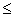k < n, we bring its transition matrix A to its canonical (or standard) form A* by listing the absorbing states first and then we make a partition of A* of the form A* =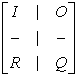, where I is the unitary k X k matrix, O is a zero matrix, R is the (n – k) X k transition matrix from the non absorbing to the absorbing states and Q is the (n – k) X (n – k) transition matrix between the non absorbing states.

If In – k denotes the unitary (n – k) X (n – k) matrix, it can be shown (e.g. , Section 2) that the square matrix In – k - Q has always a non zero determinant. Then, the fundamental matrix N of the AMC is defined to be the inverse matrix of In – k – Q. Therefore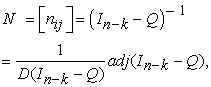(1)

where D (In – k – Q) and adj (In – k – Q) denote the determinant and the adjoin of the matrix In – k – Q respectively (, Section 2.4). We recall that the adj (In – k – Q) is the matrix of the algebraic complements of the transpose matrix of the matrix In – k – Q.

It is well known (, Chapter 3) that the element nij of the fundamental matrix N gives the mean number of times in state si before the absorption, when the starting state of the AMC is sj , where si and sj are non absorbing states.

### 4. The Markov Chain Model for PS

We introduce a finite MC on the steps Si, i=1, 2,…,5 of the PS process. Obviously this MC is an AMC with S5 its unique absorbing state. Further, it is easy to check that its transition matrix has the form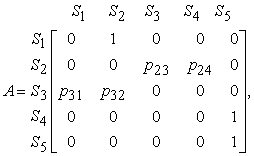with p23 + p24 = p31 + p32 = 1.

Let us denote by φ0, φ1, φ2, ...... , the successive phases of the MC and let Pi be its probability vector at phase φi , i = 0, 1, 2,.... . Then, since S1 is always the starting state, we have P0 = [1 0 0 0 0], P1 = P0 A = [0 1 0 0 0], P2 = P1 A = [0 0 p23 p24 0],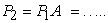(2)

It is also easy to observe that the transition matrix among the non absorbing states of the MC is equal to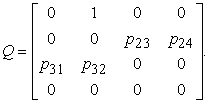Then the fundamental matrix Ν = [nij], i, j =1, 2, 3 , 4, of the chain is the matrix Ν = (I4 - Q)-1 calculated by formula (1) of Section 3. Since the element nij of Ν gives the mean number of times at state Sj before the absorption, when the MC starts from Sι and since in our case the MC starts always from S1, it becomes evident that the sum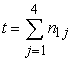gives the mean number of phases of the MC before the absorption. Performing the necessary calculations one finally finds that

Obviously, the more are the difficulties during the PS process, the greater is the value of t. Therefore, a smaller value of t is connected to a better solver performance. In other words, the value of t gives an indication of the ability either of different solver groups for solving the same problem, or of the same group for solving different problems. Of course this is not the unique indication about the difficulty of the PS process; e.g. another indication is the total time spent by the group for the completion of the PS process, etc.

### 5. Examples

The PS process of the following two problems by a group of 40 undergraduate students of the School of Technological Applications (future engineers) of the Graduate T. E. I. of Western Greece being at their first term of studies illustrates the applicability of the AMC model developed in Section 4 to real PS situations. The time allowed by the instructor for the solution of each problem was 20 minutes.

Problem 1: Given the matrix M =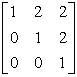and a positive integer n, calculate the matrix M n.

PS process: In the first 15 minutes 30 students solved the problem. To the rest of them the instructor gave the following hint: “Applying induction on n try to show that Mn =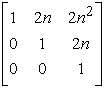”. Then six more students solved the problem in the time allowed for solution.

The transition probabilities involved in the AMC model can be calculated in this case as follows (see Figure 2): Initially all solvers proceed from S1 to S2. Then 30 of them proceed straightforward through S4 to the absorbing state S5. The other 10 solvers proceed from S2 to S3. From there, six of them return to S2 and reach S5 through S4. The remaining four solvers return to S1 and they remain there being unable to make any other movements for the problem’s solution.Download asVeiw figureFigures index
Figure 2. Flow- diagram of solver movements in Problem 1

Therefore, since we have 46 in total “arrivals” to S2 , 36 in total “departures” from S2 to S4 and 10 “departures” from S2 to S3 , one finds that p24 =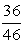and p23 =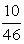. Similarly it turns out that p32 =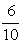and p31 =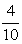.

Replacing the above values to equation (3) of Section 4 one finds that t =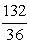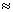3.67 steps. Also, as an example of a short run forecast, since by formulas (2) we have that P2 = [0 00], the probability for the PS process to be in its third phase at the step of executing is equal to, or approximately equal to 78.26%.

Problem 2: Let a, b, c, d be given numbers between 0 and 1. Prove that

(1- a)(1- b)(1- c)(1- d) >1- a – b – c – d (, Problem 2).

PS process: In the first 15 minutes eight students solved the problem as follows: “It is enough to show that (1- b – a + ab)(1 – d – c + cd) >1- a – b – c – d, or ab + ac + ad + bc + (bd +cd +abcd) > abc + abd + acd + bcd. But ab >abc, ac >acd, ad >abd, bc > bcd and the result follows, since bd +cd +abcd >0 “.

To the rest of the students the instructor gave the hint: “Try first to solve the corresponding problem in two and then in three variables”. Then 23 more students solved the problem in the time allowed for solution.

In this case, an argument analogous to that developed in Problem 1 shows that p24 =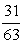, p23 =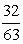, p32 =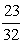and p31 =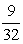and the replacement of the above values to equation (2) gives that t =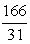5.35 steps.

Therefore, although Problem 2 involved elementary Algebra only, the students faced more difficulties than those faced in Problem 1 to solve it.

### 6. Discussion and Conclusions

In the present paper an AMC model was developed for the description of the PS process. The analysis of the PS process of the two problems of Section 5 shows that the calculation of the transition probabilities involved was based on the description of the solver assumed behavior, i.e. on how they could act and not on how they really act in practice for solving the problems. In Problem 1, for example, we have tacitly assumed that the 30 students who solved it in the first 15 minutes, proceeded linearly from S2 to S5 through S4, which could not be true. In fact, some of them could have passed from S3 first and possibly they could have made the same circle more than once. Similarly, we have tacitly assumed that the six students who failed to solve the problem proceeded for S3 to S1 and remained there until the end of the PS process, which also could not be true. In fact, some of them in their effort to solve the problem could have repeated the same circle more than once. The only way for the instructor to be helped to know if the assumed student behavior can be considered as a reasonable approach to their real behavior is to perform interviews asking the students about how they tried to solve the problems. This could be done during a research project, but it is technically difficult - due to the lack of time - to be attempted in the everyday practice, if the instructor decides to use the AMC model for evaluating the student overall performance. However, even the latter case is better providing to the instructor more information than the traditional way of marking the student papers and calculating the mean value of their marks, which is based on student final outcomes only.

They are very many other situations in Education that can be modelled by MCs, e.g. see [11, 13], etc. requiring further research. An interesting perspective for future research is also the comparison of the MC models to corresponding models developed in terms of principles of Fuzzy Logic (FL), e.g. see [13, 14], etc. In fact FL, due to its nature of characterizing the ambiguous cases with multiple values, offers the possibility of studying easier the real instead of the assumed behavior of individuals. In contrast, the fuzzy models are not so accurate like the MC ones, since the membership functions of the corresponding fuzzy sets can be defined in multiple ways according to the researcher’s personal criteria of goals. An attempt for such a comparison requires an extendable analysis, which of course could not be performed here, needing more space, plans and time. However, it is hoped to be included in our future research works.

### References

  Bartholomew, D.J. (1973). Stochastic Models for Social Processes, J. Wiley and Sons, .In article  Carlson, M.P. & Bloom, I. (2005). The cyclic nature of problem solving: An emergent multidimensional problem-solving framework, Educational studies in Mathematics, 58, 45-75.In article View Article  Kemeny, J. G. & Snell, J. L. (1963). Mathematical Models in the Social Sciences, Ginn and Company, New York, USA.In article  Kemeny, J. G. & Snell J. L. (1976). Finite Markov Chains, Springer - Verlag, New York, USA.In article  Morris, A. O. (1978). An Introduction to Linear Algebra, Van Nostrand Beinhold Company Ltd., Berkshire, England.In article  Polya, G. (1945). How to Solve it, Princeton University Press, Princeton.In article  Schoenfeld, A. (1980). Teaching Problem Solving skills, Amer. Math. Monthly, 87, 794-805.In article View Article  Schoenfeld, A. (2010). How we think: A theory of goal-oriented decision making and its educational applications; Routledge: New York.In article  Suppes, P. & Atkinson, R. C. (1960). Markov Learning Models for Multiperson Interactions, Stanford University Press, Stanford-California, USA.In article  Voskoglou, M. Gr. & Perdikaris, S. C. (1991). A Markov chain model in problem- solving, International Journal of Mathematical Education in Science and. Technology, 22, 909-914.In article View Article  Voskoglou, M. Gr. (2007). A stochastic model for the modelling process. In: Mathematical Modelling: Education, Engineering and Economics (ICTMA 12), Chaines, Chr., Galbraith, P., Blum W. and Khan, S. (Eds). 149-157, Horwood Publishing, Chichester, England.In article View Article  Voskoglou, M. Gr. (2011). Problem-Solving from Polya to Nowadays: A review and Future Perspectives, in Nata, R. (Ed.). Progress in Education, Vol. 22, Chapter 4, 65-82, Nova Publishers, New York.In article  Voskoglou, M. Gr. (2011). Stochastic and Fuzzy Models in Mathematics Education, Artificial Intelligence and Management, Lambert Academic Publishing, Saarbrucken, Germany.In article  Voskoglou, M. Gr. (2015). Defuzzification of Fuzzy Numbers for Student Assessment, American Journal of Applied Mathematics and Statistics, 3(5), 206-210.In article  Voskoglou, M. Gr. (2016). Applications of Finite Markov Chain Models to Management, American Journal of Computational and Applied Mathematics, 6(1), 7-13.In article  Voskoglou, M. Gr. (2016). Problem Solving in our Knowledge Society and Future Perspectives, in K. Newton (Ed.). Problem-Solving: Challenges and Outcomes, Nova Publishers, Chapter 13, 243-258.In article